### A Brief Guide to Engineering Financial Calculations: Interest

Key Assumptions

For each method, the interest rateis assumed to be for the interest period. For example, if the period is one year, then the interest rateis the annual interest rate; but if the interest period is one month, then the interest rate for the periodwould be 1/12 of the annual interest rate. Interest rate, discount rate, and return rate all mean the same thing.

Calculations

Direct calculation methods and interest tables were developed to solve a number of different simple cash flow series. These cash flows are idealized cases (which makes them good for midterm questions that test understanding); but in practice most cash flow series are complicated and are easier to solve using spreadsheets.

Single Payment Compound Amount: The future amount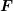at the end ofperiods after depositing a sum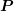at the start of theperiods, with interest rate.

In interest tables, use F = P x (F/P, i, n). The formula for direct calculation is: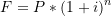(This is the same as same as the compound interest calculation in the previous section for the future value of a present sum.)

Single Payment Present-Worth Amount: The present valueequivalent to a future amountat the end of theperiods, with interest rate.

In interest tables, use P = F x (P/F, i, n). The formula for direct calculation is: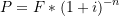Uniform Series Compound Amount: The future amountat the end ofperiods after depositing a sum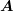at the end of each period with interest rateIn In interest tables, use F = A x (F/A, i, n). The formula for direct calculation is: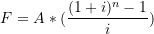Uniform Series Sinking Fund: The amountthat would have to be deposited at the end of the period forperiods with interest rateto yield the future amount.

In interest tables, use A = F x (A/F, i, n). The formula for direct calculation is: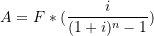Uniform Series Capital Recovery: The size of paymentsrequired at the end of each period to pay back a present sumoverperiods with interest rateIn interest tables, use A = P x (A/P, i, n). The formula for direct calculation is: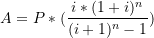Capital Recovery Cost: A related calculation is the cost to recover capital, which is

CR = (P- S)(A|P, I, N) + iS

Uniform Series Present Worth: The present sumthat would be required to invest now to provide end-of-period payments offorperiods with interest rateIn interest tables, use P = A x (P/A, i, n). The formula for direct calculation is: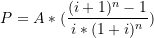Arithmetic Gradient Present Worth: The present sumfrom depositing a uniformly increasing series of sums,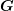,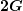, etc., for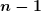periods with interest rate. Note that the sequence starts at zero payment at the end of the first period, and the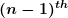payment is at the end of period.

In interest tables, use P = G x (P/G, i, n). The formula for direct calculation is: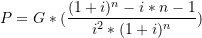Arithmetic Gradient Future Worth: The future worthat the end of n periods, from depositing a uniformly increasing series of sums,at the end of the second period,at the end of the third period, etc., to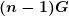at the end of period, with interest rate. Note that the sequence starts at zero payment at the end of the first period, and thepayment is at the end of period.

In interest tables, use F = G x (F/G, i, n). The formula for direct calculation is: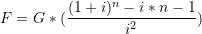Geometric Series Present Worth: The present sumfrom depositing an increasing series of sums,at the end of period 1,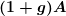at the end of period 2, etc., up to a deposit of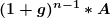at the end of periodwith interest rateIn interest tables, use P = A x (P/A, g, i, n). The formula for direct calculation is: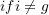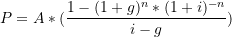otherwise: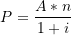Combinations

These formulæ can be used in combination to represent a more complicated set of cash flows, in a linear combination of series.

The formulæ can be used to convert from one type of series to another. One formula is used in an intermediate calculation of the equivalent(or, if you prefer), and then that value of(or) is then substituted into another equation that yields the equivalent set of payments for a different type of series, or for an equivalent sum at a different point in time.  Some examples include:

(F/P, i, N) = i(F/A, i, N) + 1(P/F, i, N) = 1 – (P/A, i, N)i

(A/F, i, N) = (A/P, i, N) – i

(A/P, i, N) = i / [1 – (P/F, i, N)]

(F/G, i, N) = (P/G, i, N)(F/P, i, N)(A/G, i, N) = (P/G, i, N)(A/P, i, N)

(F/A, g, i, N) = (P/A, g, i, N)(F/P, i, N)